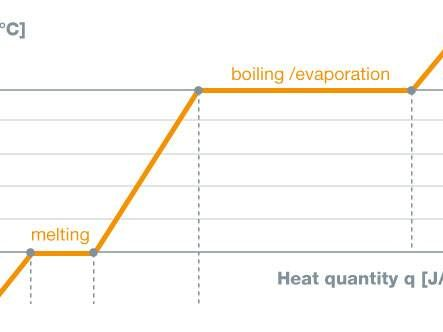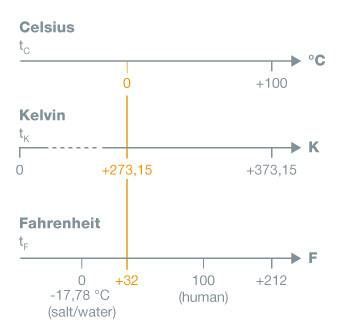# Principiile fizice

## Physical principles of the measurement parameter temperature

When a physicist speaks of temperature, he means the measure of energy inherent in a body. This energy is possessed by a body due to the random motion of its atoms or molecules. If the particles move faster, then the temperature increases. Temperature is thus a state variable. Together with mass, heat capacity and others, temperature describes the energy content of a body, or, as it often expressed in physics, a system.

Or more briefly:

• Introduction of heat energy leads to an increase of particle speed: Temperature increases
• Introduction of heat energy leads to a decrease of particle speed: Temperature decreases

When a body no longer contains any heat energy, its molecules are at rest. In reality, this state cannot be achieved. It is referred to as the absolute zero point, because there is no state with less energy. It is allocated the value 0 K (Kelvin). For this reason, Kelvin temperature is always a positive parameter.

Temperature could be directly measured in energy units. However, the expression of temperature in degrees has a long tradition, and is anchored in physics. This is why it has, for practical reasons, remained so to this day.